# Test Prep Plan - Take a practice test

Take this practice test to check your existing knowledge of the course material. We'll review your answers and create a Test Prep Plan for you based on your results.
How Test Prep Plans work
1
2Based on your results, we'll create a customized Test Prep Plan just for you!
3Study smarter
Study more effectively: skip concepts you already know and focus on what you still need to learn.

# PSAT Math - Geometry and Measurement: Tutoring Solution Chapter Exam

Exam Instructions:

Choose your answers to the questions and click 'Next' to see the next set of questions. You can skip questions if you would like and come back to them later with the yellow "Go To First Skipped Question" button. When you have completed the practice exam, a green submit button will appear. Click it to see your results. Good luck!

### Page 1

#### Question 1 1. Triangle HYV and triangle AYB are similar by the AA similarity theorem. What is the value of x?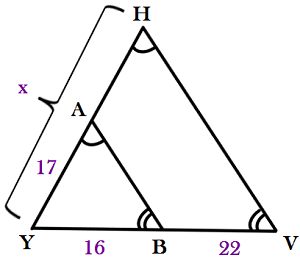#### Question 2 2. Find the shortest distance between a and b by drawing a line connecting them and using the Pythagorean Theorem.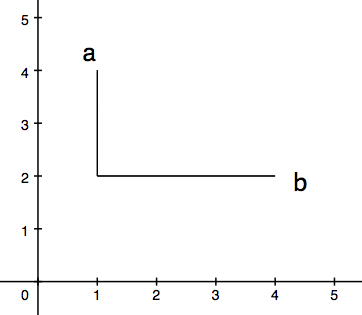#### Question 5 5. In the figure below, Angle 13 = 10y and Angle 11 = 3y + 11. Determine the measure of Angle 16.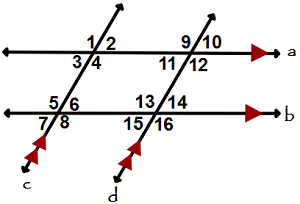### Page 2

#### Question 10 10. Jerry wants to buy a TV online, but the ad for the TV he wants to buy does not say what the screen size is. He knows that TV screens are measured diagonally. This image shows the length and width of the screen, so he decides to use the Pythagorean Theorem to determine the diagonal screen size. What is the screen size of the TV that Jerry wants to buy (to the nearest inch)?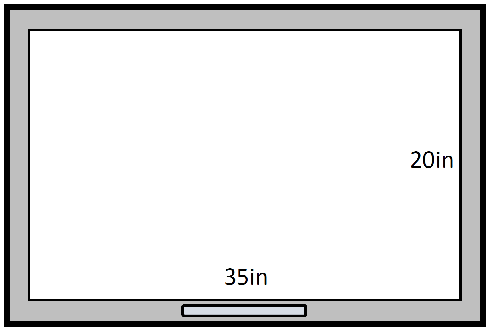### Page 3

#### Question 12 12. Consider the figure below, where Angle 4 = 12m - 4 Angle 5 = 10m + 20 Calculate the measure of Angle 5.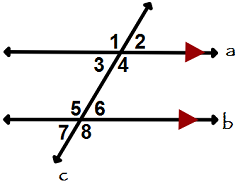#### Question 15 15. Study the given diagram. Find the value of x, the distance between T and E.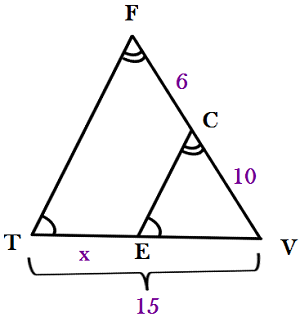### Page 4

#### Question 17 17. Which two lines are parallel?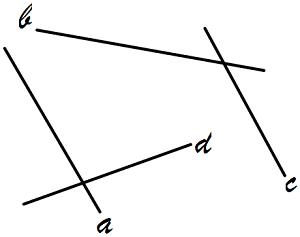#### Question 18 18. What is the relationship between Angle 1 and Angle 7?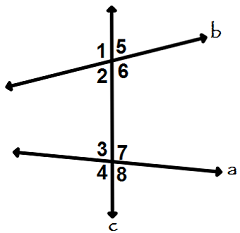#### Question 20 20. In the figure shown below, the graph of the yellow function is a translation of the graph of the blue function. If the expression for the blue function is y(x) = b(x), which of the following is the equation of the yellow function?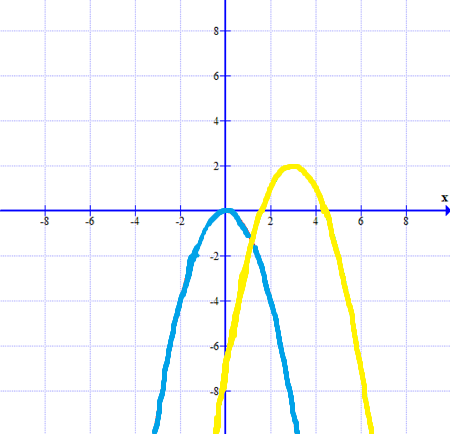### Page 6

#### PSAT Math - Geometry and Measurement: Tutoring Solution Chapter Exam Instructions

Choose your answers to the questions and click 'Next' to see the next set of questions. You can skip questions if you would like and come back to them later with the yellow "Go To First Skipped Question" button. When you have completed the practice exam, a green submit button will appear. Click it to see your results. Good luck!

Support